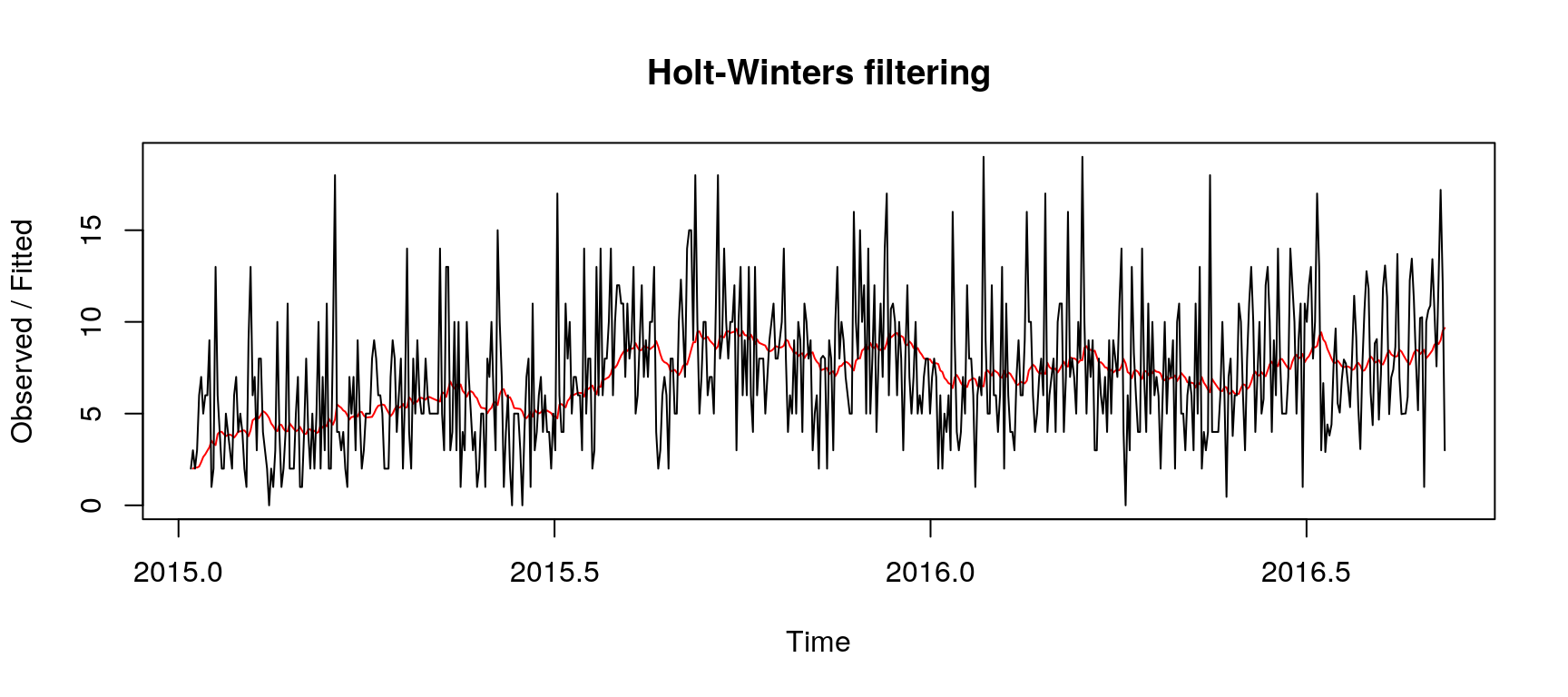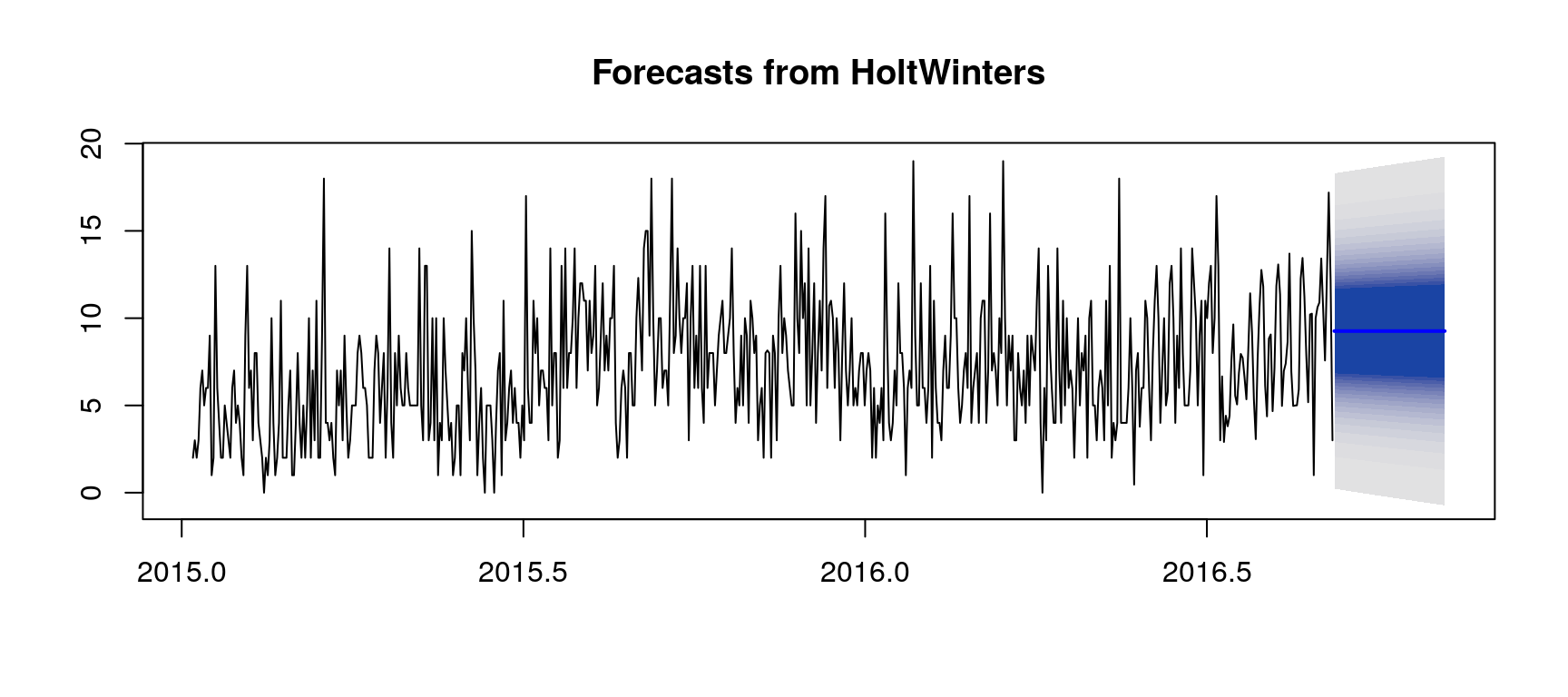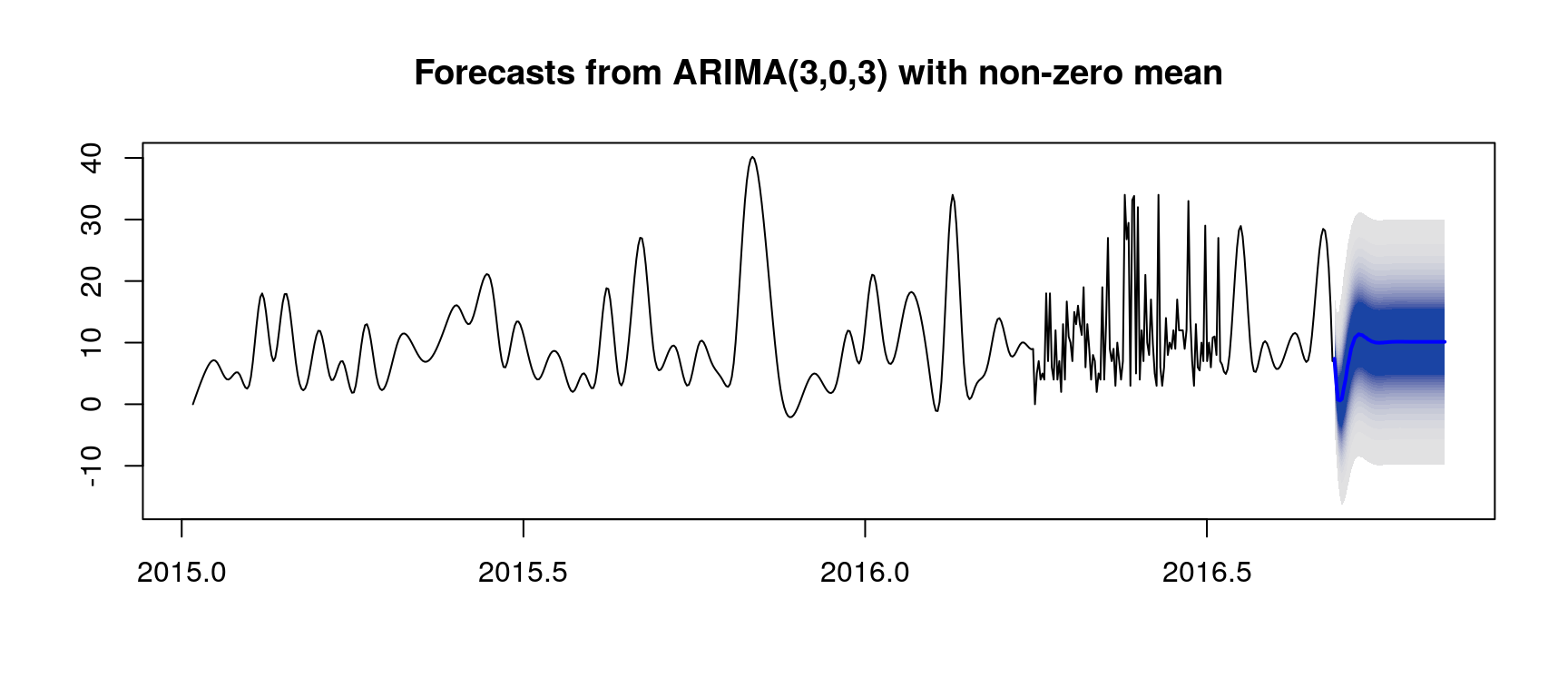Note: this post is a replication of our kernel published on Kaggle—Preliminary Investigation: HoltWinters & ARIMA.

Here we present a very simplistic, to the point of being rather naive, approach to the Kaggle’s Web Traffic Time Series Forecasting problem. Some aspects of dealing with time series in R are from A Little Book of R For Time Series (Coghlan, 2015). Each row (i.e., page) is considered individually, which might fail to capture correlations and other interactions between pages.

## Forecasting Web Traffic for the Entire Data Set

``````setnames(keys, "Page", "PageWithDate")
n <- nchar("_0000-00-00")
keys[, Page := substr(PageWithDate, 0, nchar(PageWithDate) - n)]
keys[, Date := substr(PageWithDate, nchar(PageWithDate) - n + 2, nchar(PageWithDate))]
rm (n)

# 1: ARIMA or 2: HoltWinters
kMethod <- 2
kH <- 31 + 28 + 1 # from Jan 1, 2017 to Mar 1, 2017
kUseTsClean <- TRUE
kSmoothOrder <- 0 # no smoothing if <= 0

i <- 1
forecastWebTraffic <- function(x) {
row <- unname(unlist(x))

predictions <- tryCatch({
if (kUseTsClean) {
rowTs <- tsclean(ts(row, frequency = 365.25, start = c(2015, 7, 1)))
} else {
# outliers (Hampel's test)
minMaxThreshold <- 3 * c(-1, 1) * 1.48 * mad(row, na.rm = TRUE)
+ median(row, na.rm = TRUE)
row[row < minMaxThreshold] <- NA
row[row > minMaxThreshold] <- NA

# missing values
rowTs <- ts(row, frequency = 365.25, start = c(2015, 7, 1))
if (anyNA(rowTs)) {
rowTs <- na.interpolation(rowTs, option = "spline")
}
}
if (kSmoothOrder > 0) {
smoothed <- ma(rowTs, order = kSmoothOrder)
indexes <- (kSmoothOrder %/% 2 + 1):(length(rowTs) - (kSmoothOrder %/% 2 + 1))
rowTs[indexes] <- smoothed[indexes]
rm(smoothed, indexes)
}

# predictions
if (kMethod == 1) {
fit <- auto.arima(rowTs)
} else {
fit <- HoltWinters(rowTs, beta = FALSE, gamma = FALSE)
}
predictions <- forecast(fit, h = kH, fan = FALSE)
}, error = function(e) {
predictions <- data.frame(mean = rep(0, kH))
})

i <<- i + 1
if (kIsOnKaggle && i == 100) {
stop("Stop execution to prevent time out on Kaggle", call. = FALSE)
}

return (as.list (as.numeric(predictions\$mean)))
}

vars <- names(X[, -1])
try(X[,
c(unique(keys\$Date)) := forecastWebTraffic (.SD),
.SDcols = vars, by = 1:nrow(X)])

meltX <- melt(
X[, which(names(X) %in% c(unique(keys\$Date), "Page")), with = FALSE],
measure.vars = unique(keys\$Date),
variable.name = "Date",
value.name = "Visits")
meltX\$Date <- as.character(meltX\$Date)

mergeKeysMeltX <- merge(keys, meltX, by=c("Page", "Date"), all.x = TRUE)

fwrite(
mergeKeysMeltX[, c("Id", "Visits")],
file = file.path(
".",
paste0(
"output-",
ifelse(kMethod == 1, "arima", "holtwinters"),
".csv"),
fsep = .Platform\$file.sep),
row.names = FALSE,  quote = FALSE)
``````

One can find further details about the Hampel’s test for outlier detection in Scrub data with scale-invariant nonlinear digital filters.

## HoltWinters Illustration with a Single Page

Here we consider the page 大月薰_zh.wikipedia.org_all-access_spider.

``````row <- unname(unlist(X[rowIndex, -1, with = FALSE]))
rowTs <- removeOutliersAndImputNa (row)
fit <- HoltWinters(rowTs, beta = FALSE, gamma = FALSE)
predictions <- forecast(fit, h = kH, fan = TRUE)
``````## ARIMA Illustration with a Single Page

Here we consider the page Missing9_zh.wikipedia.org_all-access_spider.

``````row <- unname(unlist(X[rowIndex, -1, with = FALSE]))
rowTs <- removeOutliersAndImputNa (row)
fit <- auto.arima(rowTs)
predictions <- forecast(fit, h = kH, fan = TRUE)
``````